• cpp #include using namespace std; class A{ public: virtual Print() { cout; } }; class B:public A{ public: Print() { cout; } }; class C:public B{ ...c++ c++
• <p>int a=6； if（a=2） printf（“%d\n”，a）； else printf（“%d\n”，a+1）； return 0；</p>c语言
• komorebi-JCE在本文您仔细讲解Java程序输出结果写入文件相关知识和一些Code实例，欢迎阅读和指正，我们先划重点：java,输出结果,java输入输出,java简单输入输出代码，下面大家一起来学习吧。1.将输出结果输出...
想了解将Java程序的输出结果写入文件方法实例的相关内容吗，komorebi-JCE在本文为您仔细讲解Java程序输出结果写入文件的相关知识和一些Code实例，欢迎阅读和指正，我们先划重点：java,输出结果,java输入输出,java简单的输入输出代码，下面大家一起来学习吧。1.将输出结果输出到txt文件直接安排代码：//导包import java.io.FileNotFoundException;import java.io.PrintStream;public class Test {public static void main(String[] args) throws FileNotFoundException {// 创建一个打印输出流，输出的目标是D盘下的1.txt文件PrintStream ps = new PrintStream("D:\\1.txt");//可能会出现异常，直接throws就行了System.setOut(ps);//把创建的打印输出流赋给系统。即系统下次向 ps输出System.out.println("看看我在哪里？");System.out.println("==============");System.out.println("我在D盘下的1.txt文件中去了。");ps.close();}}运行结果：2.将输出结果重新输出到屏幕其实代码跟前面只有一点出入。强调：在把系统的输入方向改变时，如果还要重新回到系统默认输出的话，就一定要在改变之前保存原来系统的输出方向。//导包import java.io.FileNotFoundException;import java.io.PrintStream;public class Test {public static void main(String[] args) throws FileNotFoundException {// 创建一个打印输出流，输出的目标是D盘下的1.txt文件PrintStream out = System.out;// 先保存系统默认的打印输出流缓存//一定要先保存系统最初的打印输出，不然后面就改不回来了！！！PrintStream ps = new PrintStream("D:\\1.txt");//可能会出现异常，直接throws就行了System.setOut(ps);//把创建的打印输出流赋给系统。即系统下次向 ps输出System.out.println("看看我在哪里？");System.out.println("==============");System.out.println("我在D盘下的1.txt文件中去了。");ps.close();System.setOut(out);//把打印输出流还给系统System.out.println("我又回到屏幕啦！");System.out.println("哈哈哈哈哈啊哈");}}运行结果：总结相关文章
展开全文• 问题及代码： 运行结果： 知识点总结： 学习心得：
﻿﻿
问题及代码：
/*Copyright (c)2016,烟台大学计算机与控制工程学院
*文件名称：main.cpp
*作    者：崔青青
*完成日期：2016年6月13日
*版 本 号：v1.0
*问题描述：
*输入描述：无
*输出描述：无
*/

#include <iostream>
#include<fstream>
#include<cstring>
#include<cstdlib>
using namespace std;

int main()
{
0x2B,0xBF,0xCE,0xCC,0xC3,0xCE,0xAA,0xC4,0xE3,0xB4,
0xF8,0xC0,0xB4,0xD1,0xA7,0xCF,0xB0,0xB7,0xBD,0xB7,
0xA8,0xB5,0xC4,0xB8,0xC4,0xB1,0xE4,0xA3,0xA1};
ofstream outfile("f3.dat",ios::out|ios::binary);
outfile.write((char*)a,sizeof(a));
outfile.close();
return 0;
}


运行结果：

知识点总结：
ios::out以输出方式打开，并且以二进制形式打开。
学习心得：

展开全文• main() { char *ptr = ” Cisco Systems”; *ptr++; printf(“%sn”,ptr); ptr++; printf(“%sn”,ptr); }
• 下面是当参数值31和6时的输出结果: import java.util.Scanner; public class abc { public static void main(String[] args) { Scanner sc = new Scanner(System.in); int total = sc.nextInt();//总天数
题目：
编写一个程序输出日历。该程序必须实现按下列方式输出某月信息的方法。该方法的参
数为该月的天数和第一个星期日的日期。下面是当参数值为31和6时的输出结果: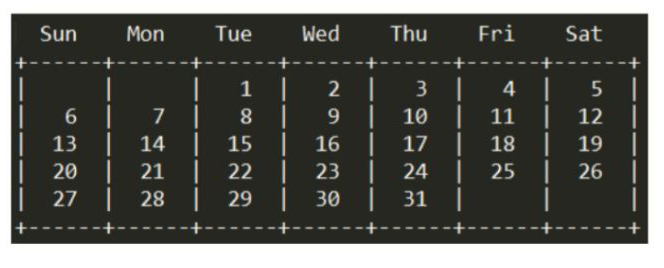import java.util.Scanner;

public class abc {
public static void main(String[] args) {
Scanner sc = new Scanner(System.in);
int total = sc.nextInt();//总天数
int day = sc.nextInt();//第一个星期日的日期

Object[] date = new Object;
date = day;

//处理前半段
int j=1;
for (int i=6;i>=0;i--){
date[i]=day-j;
j++;
if ((int)date[i]<=0){
date[i]=' ';
}
}

//处理后半段
int m=1;
for (int i=8;i<=41;i++){
if ((day+m)<=total){
date[i]=day+m;
m++;
}
}

//打印输出
System.out.println("  Sun      Mon     Tue     Wed      Thu     Fri    Sat");
System.out.println("+-------+-------+-------+-------+-------+-------+-------+");
for(int i=0;i<date.length;i++) {

if (date[i]==null){
date[i]=' ';
}
if (i==6||i==13||i==20||i==27||i==34||i==41){

System.out.println("|\t"+date[i]+"\t|");
}
else {
System.out.print("|\t"+date[i]+"\t");
}
}

System.out.println("\n+-------+-------+-------+-------+-------+-------+-------+");
}
}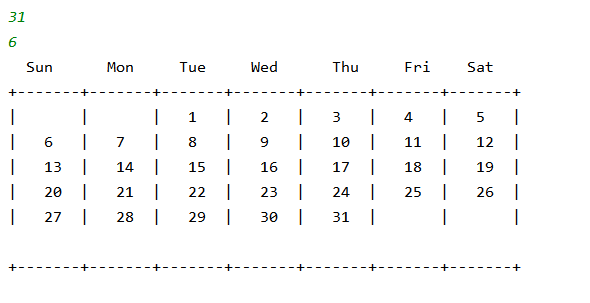展开全文java javase
• .............. void main() { float min(float x,float y); float a,b,m; scanf("%f%f",&a,&b); m=min(a,b); printf("min is %d\n",m); system("pause"); } float min(float x,float y) { float z;...}printf
• 下面小编就大家分享一篇Python3.6笔记之将程序运行结果输出到文件方法，具有很好参考价值，希望对大家有所帮助。一起跟随小编过来看看吧
• 什么写这个程序？ 由于在完成单纯形算法作业时，那么多数，对于粗心我来说，很容易做错。网上又没找到输出带过程的程序，所以只好花点时间自己写一个。 先说一下前提：一定要先转化成初始单纯形表，右端向量...
为什么写这个程序？
由于在完成单纯形算法的作业时，那么多数，对于粗心的我来说，很容易做错。网上又没找到输出带过程的程序，所以只好花点时间自己写一个。
先说一下前提：一定要先转化成初始的单纯形表，右端向量都大于等于0，并且有一个初始可行基，初始单纯形表示我们的输入。单纯形表的行数包括检验数行，列数包括右端向量，各行数据要先行后列，单纯形表的行的变量的下标要按顺序给出，列也一样。
注：程序的输出不是很美观，但这不是大问题
其他算法的计算可以看我的另一个博文：山东大学软件学院最优化考试复习笔记
先看下面的一个例子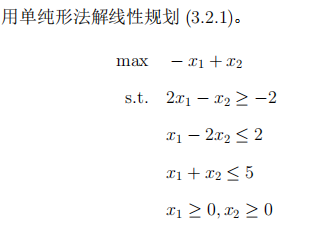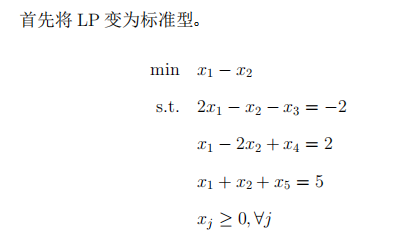初始单纯性表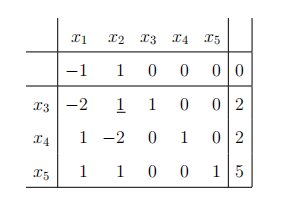继续算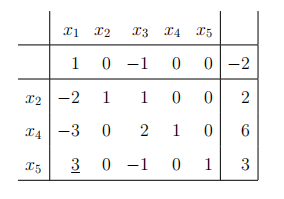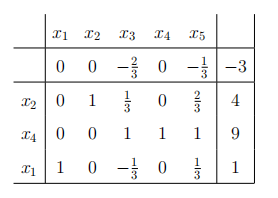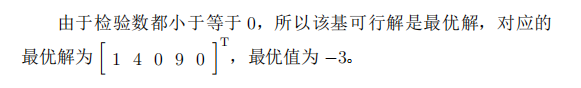进基是按照最大检验数规则和最小下标原则。
输出的第一个表是输入的初始单纯形表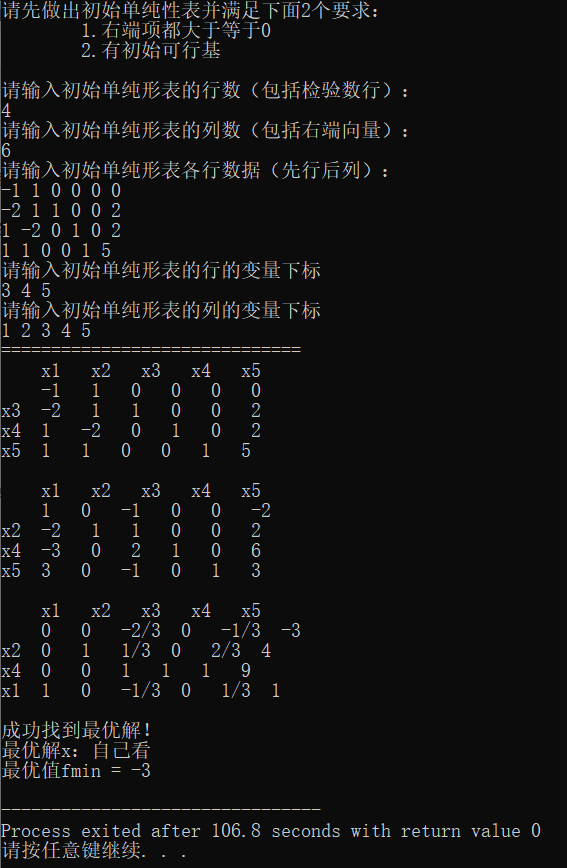#include <iostream>
using namespace std;

int row, column; // row是初始单纯形表的行数，column是初始单纯形表的列数

struct Fraction // 分数
{
int up, down = 1; // 分子，分母
};

Fraction table; // 单纯形表

int inBase(); // 返回进基变量下标
int outBase(int); // 返回出基变量下标
void showTable(); // 显示当前单纯形表
Fraction reduction(Fraction); // 化简分数
Fraction minu(Fraction, Fraction); // 分数相减
Fraction multi(Fraction, Fraction); // 分数相乘
Fraction divide(Fraction, Fraction); // 分数相除
int abs(int); // 返回绝对值
int gcd(int, int); // 返回最大公约数

int main()
{
cout << "请先做出初始单纯性表并满足下面2个要求：" << endl;
cout << "	1.右端项都大于等于0\n	2.有初始可行基" << endl << endl;
// 处理输入
cout << "请输入初始单纯形表的行数（包括检验数行）：" << endl;
cin >> row;
cout << "请输入初始单纯形表的列数（包括右端向量）：" << endl;
cin >> column;
cout << "请输入初始单纯形表各行数据（先行后列）：" << endl;
for (int i = 1; i <= row; i++)
{
for (int j = 1; j <= column; j++)
{
cin >> table[i][j].up;
}
}
cout << "请输入初始单纯形表的行的变量下标" << endl;
for (int i = 2; i <= row; i++)
cout << "请输入初始单纯形表的列的变量下标" << endl;
for (int i = 1; i <= column-1; i++)
cout << "==============================" << endl;
showTable();
cout << endl;

int inVar = inBase(); // 计算进基变量
int outVar; // 出基变量
int isOk = 0; // 判断是否已经结束，0未结束，1找到最优解，2问题无界
while (!isOk)
{
if (inVar == 0)
{
// 满足最优性准则，此时为最优解，停止
isOk = 1;
}
else
{
outVar = outBase(inVar); // 计算出基变量
if (outVar == 0)
{
// 此时问题无界，停止
isOk = 2;
}
}

if (!isOk)
{
// 按照算法进行操作
Fraction temp = table[outVar][inVar];
for (int j = 1; j <= column; j++)
table[outVar][j] = divide(table[outVar][j], temp);

for (int i = 1; i <= row; i++)
{
if (i == outVar)
continue;
else
{
temp = table[i][inVar];
temp.up = -temp.up;
for (int j = 1; j <= column; j++)
}
}
showTable();
inVar = inBase();
cout << endl;
}
}

if (isOk == 1)
{
cout << "成功找到最优解！" << endl;
cout << "最优解x：自己看" << endl;
cout << "最优值fmin = ";
if (table[column].down == 1)
cout << table[column].up << endl;
else
cout << table[column].up << "/" << table[column].down << endl;
}
else
{
cout << "问题无界！" << endl;
}

return 0;
}

int inBase()
{
// 选择进基变量
// 进基变量按最大检验数规则和最小下标原则
int result = 0; // 保存最大变量的下标
for (int j = 1; j <= column-1; j++)
{
if (table[j].up > 0)
{
if (result == 0)
result = j;
else if (minu(table[j], table[result]).up > 0)
result = j;
}
}
return result;
}

int outBase(int num)
{
// 选择出基变量（num是出基变量所在的列，也是进基变量所在的列）
// 出基变量根据最小比值测试结果和最小下标原则
int result = 0; // 保存最小比值测试中最小的下标
Fraction temp[column+1]; // 保存计算结果
for (int i = 2; i <= row; i++)
{
if (table[i][num].up > 0)
{
if (result == 0)
result = i;
temp[i] = divide(table[i][column], table[i][num]);
if (minu(temp[i], temp[result]).up < 0)
result = i;
}
}
return result;
}

void showTable()
{
// 输出结果
cout << "    ";
for (int i = 1; i <= column-1; i++)
cout << "x" << head[i] << "   ";
cout << endl;
cout << "    ";
for (int j = 1; j <= column; j++)
{
if (table[j].down == 1)
cout << table[j].up << "   ";
else
cout << table[j].up << "/" << table[j].down << "  ";
}
cout << endl;
for (int i = 2; i <= row; i++)
{
cout << "x" << answer[i] << "  ";
for (int j = 1; j <= column; j++)
{
if (table[i][j].down == 1)
cout << table[i][j].up << "   ";
else
cout << table[i][j].up << "/" << table[i][j].down << "  ";
}
cout << endl;
}
}

Fraction reduction(Fraction result)
{
// 化简分数
// 1. 使down为非负数。如果分数为负，那么令分子up为负即可
// 2. 如果该分数恰为0，那么规定其分子为0，分母为1
// 3. 分子和分母没有除了1以外的公约数
if (result.down < 0)
{
// 如果分母为负数，那么令分子和分母都为相反数
result.up = -result.up;
result.down = -result.down;
}

if (result.up == 0)
{
result.down = 1;
}
else
{
int d = gcd(abs(result.up), abs(result.down));
result.up /= d;
result.down /= d;
}

return result;
}

{
// 分数加法，返回最简负数
Fraction result;
result.up = f1.up * f2.down + f2.up * f1.down;
result.down = f1.down * f2.down;
return reduction(result);
}

Fraction minu(Fraction f1, Fraction f2)
{
// 分数减法，返回最简分数
Fraction result;
result.up = f1.up * f2.down - f2.up * f1.down;
result.down = f1.down * f2.down;
return reduction(result);
}

Fraction multi(Fraction f1, Fraction f2)
{
// 分数乘法，返回最简分数
Fraction result;
result.up = f1.up * f2.up;
result.down = f1.down * f2.down;
return reduction(result);
}

Fraction divide(Fraction f1, Fraction f2)
{
// 分数除法，返回最简分数
Fraction result;
result.up = f1.up * f2.down;
result.down = f1.down * f2.up;
return reduction(result);
}

int gcd(int a, int b)
{
return !b ? a : gcd(b, a%b);
}

int abs(int a)
{
if (a < 0)
return -a;
else
return a;
}



展开全文单纯形算法
• |||97b/n 一个叫输出%c（字符） +3298；但是一个叫输出%d（整形变量） 加上3297；‘B’ASCLL码66 输出97和b因为这题算得是ASCLL码值；‘A’ASCLL码65 所以 输出 97和bc
• //我调试到这边查看*p_end 内容并不是最后一个字符k,问题应该就在这边？？？？ for(p_start; p_start < p_end; p_start++,p_end--) { str_tmp = *p_start; *p_start = *p_end; *p_end = str_...c语言
• 例如，下面这段程序，我如何测试他打印结果呢？ ` package com.cn.gao; import java.awt.Graphics; import java.awt.print.Book; import java.awt.print.PageFormat; import java.awt.print.Paper; import ...java java-ee
• 【填空题】要使用标准I/O库函数,必须在程序的开头包含__________文件。 (4.0分)【填空题】有以下程序: #include void main(){ char cl,c2,c3,c4,c5,c6; scanf("%c%c%c%c",&c1,&c2,&c3,&c4); c5=...
• Python3.6笔记之将程序运行结果输出到文件方法更新时间：2018年04月22日 14:27:32 投稿：jingxian下面小编就大家分享一篇Python3.6笔记之将程序运行结果输出到文件方法，具有很好参考价值，希望对大家有所...
• /*********************************************************你能猜出这段程序的输出结果吗？许多人都坚信，只要有源代码，他们就总能搞懂代码里所有玄机。下面这几行C语言代码就是对这一类人最大嘲弄：这只是...语言 编译器 c
• n 程序运行结果示例： 当公式最后一项绝对值小于n时停止计算:0.00006 PI=3.141713 输入提示："当公式最后一项绝对值小于n时停止计算:" 输入格式："%f" 输出格式ÿ...c语言
• 下面是一个Applet程序程序的功能输出一个彩色窗体。...程序的输出结果为：import java.awt.*;import java.awt.image.*;import java.applet.Applet;public class MemoryImage extends Applet{Image...
• 写出下面代码的输出结果什么？public class Test2{ static int i=5; public static void main(String[] args){ Father father = new Child(); father = new Child(); System.out.println( (chang
• 修改后的程序： #include using namespace std; class Date { public: Date(int=1,int=1,int=2005); void display(); private: int month; int day; int year; }; Date::Date(int m...
• 下面程序的输出结果是____ A:11,10 B:11,11 C:10,10 D:10,11 int x=10; int y=x++; printf("%d,%d",(x++,y),y++); 输出结果为A (y++优先级比较高，所以先计算y++，再计算（）里面内容) 转载于:...
• 代码： 1 import java.util.*; 2 public class Main { 3 public static void main (String [] args) { 4 Scanner s=new Scanner(System.in); 5 double d1=s.nextDouble(); ...
• #include<stdio.h>...PS：下面程序执行结果，上面是文字版，想要文字版朋友可以直接复制。 如果有疑问欢迎评论咨询，想要其他方法也可以哦 如果帮助到你，点个赞再走呗，可爱的朋友们 ...经验分享 c语言 算法
• ps -ef | grep *** ps [选项] 下面对命令选项进行说明： -e 显示所有进程。 -f 全格式。 -h 不显示标题。 -l 长格式。...-w 宽输出。...a 显示终端上的所有进程，包括其他用户的进程。...ps-ef显示出的结果...linux命令
• 正确答案： B本题解析：本题考查的是 Java 的基本数据类型及其运算。...Java 语言规定， char 型数据可以自动转换成 int 类型， 转换的结果就是该字符的 ASCIl 码值。因此，整型变量 aa 的初始值字符” h”的...
• 下面会有代码帮助大家理解：在swich中会先执行完所有的case后才会执行default,其次如果case或default成立后，可其后并没有bredk语句，那么他会输出其后所有能输出的语句直到遇见break或者程序到底，这里也许有同学会...c语言 经验分享struct
• 模块返回一个函数foo、一个变量bar ="stringBar" 函数执行时候，打印：fnFoo，同时返回：foo 所以上述代码，输出： fnFoo foo stringBarjs...